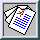Physics of Fluids A -- January 1991 -- Volume 3, Issue 1, pp. 188-200

### Simulation of the Kolmogorov inertial subrange using an improved subgrid model

Jeffrey R. Chasnov
NASA Goddard Institute for Space Studies, New York, New York 10025

(Received 12 June 1990; accepted 13 September 1990)

A subgrid model is developed and applied to a large-eddy simulation of the Kolmogorov inertial subrange. Currently popular subgrid models are derived from models of the turbulent energy equation, resulting in a significant loss of information as a consequence of the statistical averaging performed in going from the Navier-Stokes equation to the energy equation. The subgrid model developed here is based directly on a model of the Navier-Stokes equation. The improved subgrid model contains two terms: an eddy viscosity and a stochastic force. These terms are computed from the EDQNM stochastic model representation of the momentum equation, and from a fully resolved direct numerical simulation. Use of the subgrid model in a forced large-eddy simuation results in an energy spectrum that exhibits a clear k -5/3 power-law subrange with an approximate value Ko=2.1 of the Kolmogorov constant.

Full Text: [PDF (1.7 MB) GZipped PS(1.5 MB)]